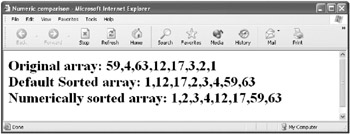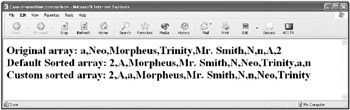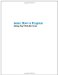# Making Weird Comparisons

 Advanced As I mentioned a little while ago when I described the sort method of the Array object, the default sort is alphabetical, from A to Z and from a to z, meaning that all the uppercase letters come before the lowercase letters. (Numbers come before either uppercase or lowercase letters in the default sort.)

This default sort may be the wrong sort of sort for you, and it’s not at all weird to need a different kind of sort in your array-processing code. Fortunately, this can be achieved by supplying the sort method a custom comparison function. With a custom comparison function, I can sort my way!

Here’s how it works: The sort method takes as an optional argument a function that specifies how to do the comparisons for the sort (if one isn’t going to accept the default comparison). (For more about how functions work, see Chapter 5, “ Understanding Functions.”)

The function used for the custom comparison must accept two arguments, which represent two elements being compared. If the first argument (element) should appear before the second argument (element) in the sort, then the function needs to return a positive number.

On the other hand, if the first argument should appear after the second in the sorted array, then the function should return a negative number.

 Tip It’ll be easiest to wrap your brain around these comparison functions if you think of the process as a kind of game, or puzzle, to figure out!

If the two values are the same in the sort order, then the function should return 0.

Table 6-5 shows these rules for a hypothetical custom sort function that accepts the arguments a and b as representing the elements in an array.

Table 6-5: Return Values for a Custom Sort Function That Accepts the Arguments a and b

Sort Evaluation

Function Return

a > b

Positive number, for example, 1

a < b

Negative number, for example, –1

a = b

0

This is really a lot less difficult than it sounds, as you’ll see in the context of a couple of practical examples. Coming up with sort functions is a lot of fun if you think of it as a kind of game. That’s why I ask you at the end of this section to invent your own sort functions!

### Sorting Numerically

The first example I show you will sort the elements of an array in numerical order. This is quite different from alphabetic order in JavaScript. Take the pair of numbers 17, 2 to understand why. Clearly, sorting them numerically would produce 2, 17. But the standard alphabetic sort converts each numeral in 17 to a string, and the first numeral (1) is ahead of 2 in alphabetic order. So the standard sort for the pair stays at 17, 2.

Here’s a function that provides the correct returns, as shown in Table 6-5, for a numerical sort:

` function numSort(a,b){      return a - b;  } `

Think about it: If you pass this function the 17, 2 pair, it’ll return a positive number (17 – 2 = 15). Returning a positive number means that 17 is greater than 2, so the pair will be correctly ordered 2, 17. You can also easily see that this function would return the correct result (a negative number) if the first argument passed to it were less than the second; for example, the pair 2, 17 would return –15, so the pair would be ordered from smallest number to highest.

Let’s use this function in a small program that first populates and displays an array of numbers:

` var numArray = new Array (59, 4, 63, 12, 17, 3, 2, 1)  document.write ("Original array: " + numArray); `

Next, let’s display the array the way it would be sorted with the default Array.sort method:

` document.write ("Default Sorted array: " + numArray.sort()); `

The default-sorted array appears as 1,12,17,2,3,4,59,63. Although it’s easy to see how this ordering was arrived at (I explained it a little earlier), the ordering could give your program fits and starts if it was expecting a numerically sorted array.

Finally, display the array sorted numerically using our custom function:

` document.write ("Numerically sorted array: " + numArray.sort(numSort)); `

This time the array is correctly sorted from lowest to highest: 1,2,3,4,12,17,59,63.

There, that was easy (and useful, too)! Figure 6-13 shows the display generated by this code, with the complete HTML page that includes the code shown in Listing 6-11.

Listing 6.11: Custom Numeric Comparison for the Array.Sort Method` <HTML> <HEAD> <TITLE>  Numeric comparisons  </TITLE> <HEAD> <BODY> <H1> <SCRIPT>      function numSort(a,b){         return a - b;      }      var numArray = new Array (59, 4, 63, 12, 17, 3, 2 , 1)      document.write ("Original array: " + numArray);      document.write ("<br>");      document.write ("Default Sorted array: " + numArray.sort());      document.write ("<br>");      document.write ("Numerically sorted array: " +         numArray.sort(numSort));     </SCRIPT> </H1> </BODY> </HTML> `Figure 6-13: Default sort comparison and custom numeric comparison

### Performing a Case-Insensitive Sort

Suppose you’d like to do a case-insensitive sort. This means that the sort evaluates lowercase and uppercase (capital) letters in the same way. If you compare a big A with little a, they should evaluate as equal. This is very much not the case for the default sort order, in which all the uppercase letters (up to Z) come before the first lowercase letter (a).

Case-insensitive sorting is something that comes up often in the course of programming. It’s pretty easy to do using String object methods. However, I don’t want to put the cart before the horse.

Working with strings is an important topic for most programmers. It’s the subject of entire chapter in this book, Chapter 9, “ Working with Strings.” There’s just enough about it here to show you how to implement the case-insensitive string comparison. If you want to delve more deeply into strings, please turn to Chapter 9.

 Note You’ll find lots of information about working with strings in Chapter 9, “ Working with Strings.”

First, let’s create, populate, and display a string array:

` var theArray = new Array("a","Neo","Morpheus","Trinity",     "Mr. Smith", "N", "n", "A",2                              );  document.write ("Original array: " + theArray); `

Next, let’s display the array the way it would be sorted with the default

Array.sort method:

` theArray.sort();  document.write ("Default Sorted array: " + theArray); `

Here’s the code that performs the case-insensitive sort using a custom function and displays the results:

` theArray.sort(function(x,y){     var a = String(x).toUpperCase();     var b = String(y).toUpperCase();     if (a > b)        return 1     if (a < b)        return -1     return 0;  });  document.write ("Custom sorted array: " + theArray); `

Figure 6-14 shows the display generated by this code, with the complete HTML page that includes this code shown in Listing 6-12.

Listing 6.12: Case-Insensitive Comparison for the Array.Sort Method` <HTML> <HEAD> <TITLE>  Case-insensitive comparison  </TITLE> <HEAD> <BODY> <H1> <SCRIPT>     var theArray = new Array("a","Neo","Morpheus","Trinity",        "Mr. Smith", "N", "n", "A",2);      document.write ("Original array: " + theArray);      document.write ("<br>");      theArray.sort();      document.write ("Default Sorted array: " + theArray);      document.write ("<br>");      theArray.sort(function(x,y){        var a = String(x).toUpperCase();        var b = String(y).toUpperCase();        if (a > b)           return 1        if (a < b)           return -1        return 0;      });      document.write ("Custom sorted array: " + theArray);     </SCRIPT> </H1> </BODY> </HTML> `Figure 6-14: Default sort comparison and case-insensitive comparison

A couple of words about the function I just showed you: First, this function is never named and appears in place in code. There’s no real need to name it because it’s used only once. A function used this way is called a function literal, or sometimes a lambda function.

Second, if you look at what the function does, it converts each of the two inputs explicitly to the String type using the String function. The String object’s toUpperCase method is then used to convert both input strings to all upper case so that a case-insensitive comparison can be performed. Normal string comparison is then used to see which, if either, uppercase string “is greater” than the other, and the appropriate positive, negative, or 0 value is returned.

I’ve shown you two custom sort functions for use with the Array.sort method: sorting numerically and using a case-insensitive string sort. The best way to learn is by doing.

Now that you know how to do weird comparisons, can you create your own interesting custom sort functions?Learn How to Program Using Any Web Browser
ISBN: 1590591135
EAN: 2147483647
Year: 2006
Pages: 115
Authors: Harold Davis

Similar book on Amazon